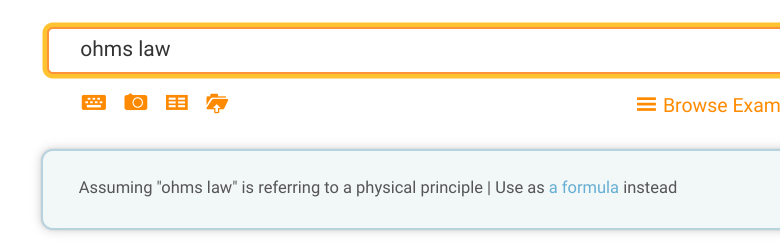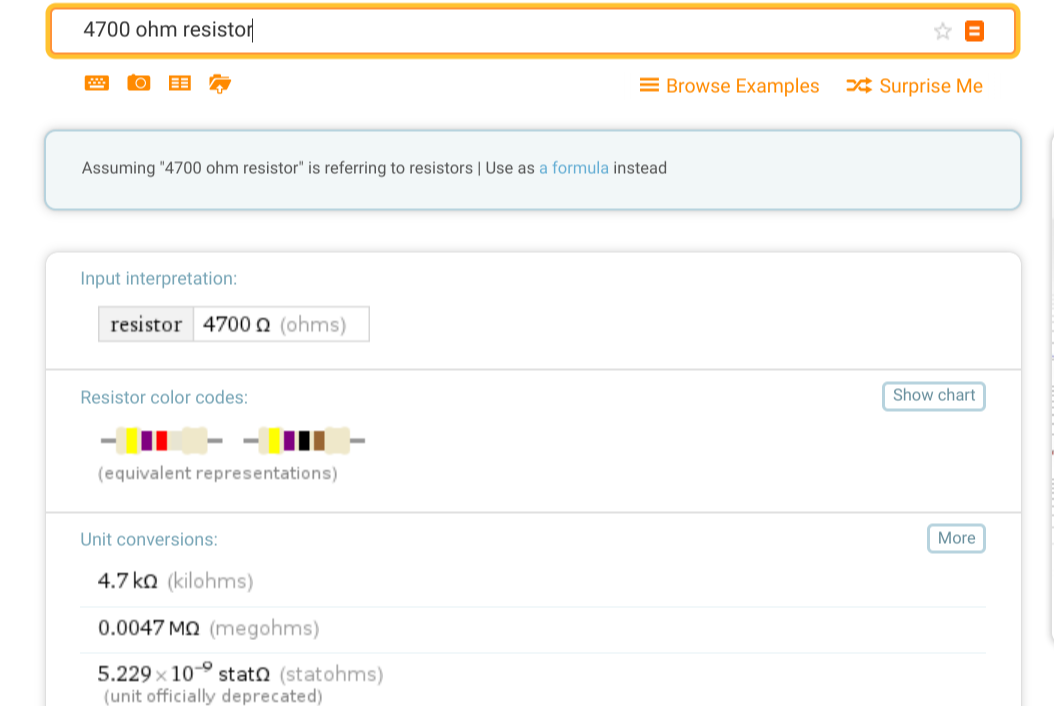# Wolfram Alpha Electronic TipsElectronics takes a lot of math. Once you’ve mastered all the algebra and calculus, though, it is sometimes a drag to go through the motions. It also can be error-prone. But these days, you have Wolfram Alpha which will do all the work for you and very easily. I use it all the time when I’m too lazy to solve an equation or do an integral by hand. But did you know it actually has some features specifically for electronics?

If you want to do a lot with electronics — or nearly any technical field — you are going to have to learn some math and you shouldn’t just rely on tools like Wolfram to skirt understanding the math. Unfortunately, schools often teach us that the point to math is to get a correct answer. For bookkeepers and at the very final stage of engineering, that may be true. But the real value to math for engineers and scientists is to develop intuition about things. If you increase a capacitor’s value does that make its reactance go up or down? Does a little change in load resistance make a corresponding small change in power consumption or is it a lot more? So you should understand why math works. But once you do, using a tool like Wolfram can free you to focus on the abstract questions instead of the detailed “grunt work.”

## Tip #1: Split Personality

Wolfram can’t seem to decide if it is a symbolic math program or a search engine. Sometimes just putting a topic name in can lead to some interesting calculations. For example, look what happens when you enter the word opamp:You get quite a few options of different types of opamp circuits and how to analyze them. Wolfram is pretty good at picking up suffixes like mV for millivolt, although we’ve found a few that choke it (see tip #2). Other topics you might find interesting are various combinations of parallel or serial RLC circuit — “serial LC circuit,” for example. Entering “Ohm’s law” tells you about George Ohm, but entering “Ohm’s law calculator” is a bit more practical. If you forget, though, you can always click the formula link:Try “Butterworth filter” or “Chebyshev filter.” Unfortunately, “Sallen-key filter” or “Elliptic filter” don’t do anything useful.

## Tip #2: Quick Calculations

You can often give the site a keyword and some parameters and it will sort them out. For example, if you need a capacitor to resonate with a 100 uH inductor at 4 MHz:The only problem is, sometimes it gets confused about units. For example, it doesn’t pay to try to use picofarads with Wolfram:You can always express it in different units or — oddly — if you put the 100 uH in first, it will correctly figure out picofarads.

Update: As noted in the comments, the reason it was confused is it is case sensitive and the correct entry is pF. The second example working wasn’t due to the order, it was because I randomly capitalized the F in that case. Now who uses pf as per fortnight is probably another question for another day.

## Tip #3: Color Codes

You really should learn the resistor color codes. There are lots of sentences to help you remember like “Big Boys Race…” or “Bad Beer Rots…” However, if you haven’t learned them yet, you can ask Wolfram to go either direction. Try “4700 ohm resistor” or “Resistor red red orange,” for example.Of course, that might lead you down the rabbit hole of asking what is a statohm? Which will then lead to the abohm and other things too terrible to contemplate. Of course, you can always ask for “parallel resistors” and solve for the equivalent value or find a pairing that will give you a desired value.

## Tip #4: Engineering Units Solutions

Of course, Wolfram is mostly about solving equations and doing calculus. For example, suppose you have a twelve-volt battery and you want a voltage divider that produces eight volts with no load. You have the top resistor, R1, set to 100 Ω. You can ask “8=12*(R_2/(R_1+R_2)), R_1=100, solve for R_2” which works and is an easy way to set variables like R_1 for R1. You can also use things like R1, although sometimes it gets confused when you do that with larger numbers. However, what follows the underscore must be a number. So you could make a much longer query that was more symbolic.This is fine, and you could use the parallel resistor calculator to figure out a value of R2 with a given load. For example, if the load were 333 Ω (about 24 mA load), the real value of R2 would need to be about 500 Ω. If you want to double check that math, you can.

The problem is when you get a small number, it often comes back in scientific notation. Suppose you didn’t know that Wolfram has all the reactance calculators built-in. You know an inductor has a reactance of 2*pi*f*L, and you want to know what inductor would have a reactance of 100 Ω at 122 MHz.

This is easy to set up (I used x instead of L to prevent the site from getting confused about units). You can use E to represent a power of 10, so 122E6 is 122 MHz. Initially, it will tell you the answer is a fraction, but you can press the “Approximate form” button to get a decimal answer. The problem is, that will show zero until you press “More digits.” The result is 1.305 x 10-7 H. So is that 0.13 μH Or 130 nH? You can ask Wolfram to do it for you. But it is hit and miss if you can ask it to just produce the answer in engineering units all in one query.

## Tip #5: It Works With Computer Stuff, Too

If you are more into the computer side of electronics, Wolfram can help with that too. Need Unicode characters? Need an MD5? Maybe hex, decimal, binary, and octal conversions? You can even get information about IP addresses and ranges.

I guess the point is, that while you think of Wolfram as math solver, it knows a lot about many things including lots of branches of engineering. Perhaps the frustrating thing about it is that while it knows a lot, it doesn’t know everything. So for every opamp calculator in it, there’s a missing one for voltage dividers or the specific filter you need.

Still, it is a great timesaver. If you understand the math, you can set up some pretty interesting equations and solve them. If you don’t understand the math, you can use the site to see how it answers certain questions, which is useful, too.

## 13 thoughts on “Wolfram Alpha Electronic Tips”

1. Clara says:

I also find GNU Units is an invaluable tool for engineering calculations: a scientific calculator that keeps track of, and converts, units.

2. Digital Corpus says:

3. Ostracus says:

“If you increase a capacitor’s value does that make its reactance go up or down? Does a little change in load resistance make a corresponding small change in power consumption or is it a lot more?”

SPICEing up the subject.

4. Taylor Street says:

The units for picofarads works fine if you just put them in properly as “pF” instead of “pf.”

1. TheRegnirps. says:

+1

5. Pat says:

“The only problem is, sometimes it gets confused about units. For example, it doesn’t pay to try to use picofarads with Wolfram:”

That’s because picofarads is pF, not pf. If you put in pF, it works fine. I have no idea why “pf” is an abbreviation for “per fortnight” on Wolfram Alpha, but it is – and since ‘per fortnight’ is a frequency, it thinks you’re asking for the capacitance for a given frequency and inductance.

“You can always express it in different units or — oddly — if you put the 100 uH in first, it will correctly figure out picofarads.”

That’s because you used pF there and not pf. If you use pf there, it still thinks you mean per fortnight.

1. Al Williams says:

Ah good catch. I am so used to Spice which gets upset because 5M is 5 milli upper or lower case (use 5Meg). I couldn’t figure out why it wanted fortnights.

6. Mbella says:

I use to use wolfram to quickly test truth tables and make sure i didnt mess up my boolean algerbra

1. Pat says:

Not sure which one you’re looking for, but it has plenty of them. Ask it for “distance 9.8 m/s^2 acceleration for 10 seconds”, and it’ll tell you 490 meters. Ask it for “projectile range 45 degrees 7.0 m/s” and it’ll tell you “4.997 meters”. It has the math for more complicated ones as well (elastic/inelastic collisions, etc.) but I don’t know how you’d enter it as a single search term.

7. RFP-A says: